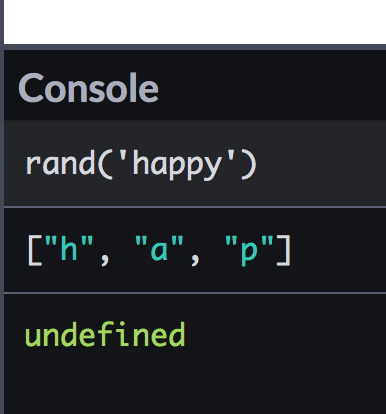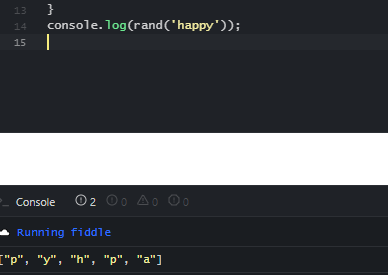# Trouble with basic function to scramble word

I’m trying to write a basic function to “scramble” (rearrange the letters of) a word. My thinking was to:

1. take the word as an argument
2. use a loop to assign the string to an array
3. use another loop to go through the array, randomly picking items from the array and pushing them to another array, and then splicing those items from the original array
4. repeating that loop until all the items have been spliced (and thus the loop stops)
5. logging the new “randomized” array to the console

I’m realizing that a `while` loop might have been a better choice to accomplish this, but to start with, this idea is not working, so I’m wondering why this isn’t functioning:

``````function rand(word) {
const wordArr = [];
const newArr = [];
for (i = 0; i < word.length; i++) {
wordArr.push(word[i]);
}
for (j = 0; j < wordArr.length; j++) {
let pick = Math.floor(Math.random() * wordArr.length);
newArr.push(wordArr[pick]);
wordArr.splice(pick, 1);
}
console.log(newArr);
}
``````

Here’s an example of what the console says.What you can try is instead of using wordArr.length

`````` for (j = 0; j < wordArr.length; j++)
``````

Use word.length

`````` for (j = 0; j < word.length; j++)
``````

because the problem is that you’re asking for the second loop to grab a not finished array from the first loop because the first loop will run and then the second one will run until the second one is done, then the first one can go again.there is the result.

1 Like

What’s `j` and why are you incrementing it in this example?

Btw, strings are iterable objects, so you can do this:

``````const wordArr = [...word];
``````
1 Like

Owh, I didn’t even noticed that he didn’t declare those variables.I’m using j as a counter to iterate through the letters of the argument `word`

But you still have to declare it as a variable. You can use let so it become a local block variable. ex:

``````for (let j = 0; j < word.length; j++) {}
``````

You still have to declare it as a variable

1 Like

This will turn the string into an array?

@Catalactics Thanks I see that error now. But why did it still work?

@El_Escandalo, I don’t really know how it works, I tried it on a different code and it also works, I don’t understand , maybe its just a built in feature? But still always declare it as a variable.

1 Like

Yes, it is saying “take the characters of this string and insert them one by one into a new array”.

Normally-used shuffle for arrays:

Example implementation in JS here:

1 Like

As far as I understand you need to iterate through the array until its length is greater than `0`, if you’re incrementing `j` this condition will break.

This will be sufficient enough for such loop:

``````for (; wordArr.length > 0;) { /* This is totally valid weird JS */ }
``````

Of course this way would be somewhat more readable and less confusing:

``````while (wordArr.length) { /* splice here */ }
``````
1 Like